# SMALL Function

Returns the k-th smallest value in a given dataset

## What is the SMALL Function?

The SMALL Function is categorized as an Excel Statistical function. It will return numeric values based on their position in a list, ranked by value. Essentially, it calculates and returns the k smallest value in a dataset.

In financial analysis, the SMALL function can be useful in finding the smallest value in a given set of values. For example, if all salespersons are given the same target, we can find out which one achieved the sales target in the shortest time for a given year.

### Formula

=SMALL(array,k)

The SMALL function uses the following arguments:

1. Array (required argument) – This is an array or range of numerical data for which we want to find the k smallest value.
2. K (required argument) – This is the smallest value from the given array.

### How to use the SMALL Function in Excel?

To understand the uses of the SMALL function, let’s consider a few examples:

#### Example

Suppose we are given the following data about several runners, along with their start and finish times: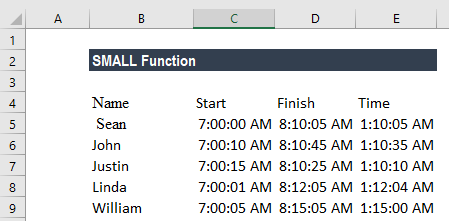We can use the SMALL function to retrieve the nth lowest value from a set of data, that is, the first, second, or third fastest times in a race.

The formula to be used in finding the winner will be: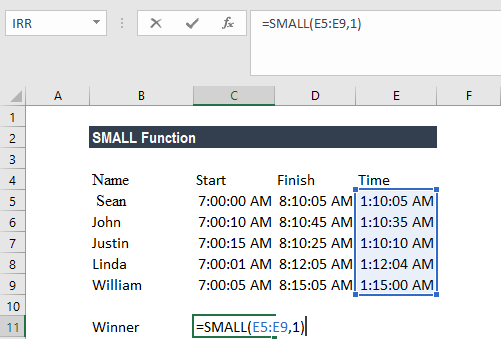The formula to be used in finding the first runner-up will be: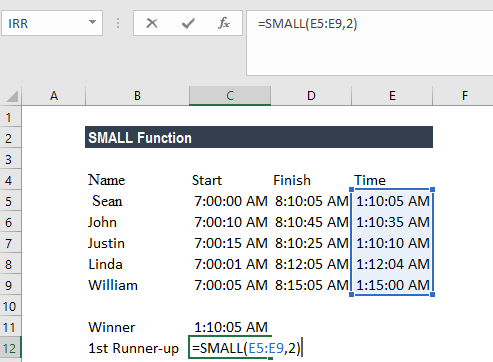The formula to be used in finding the second runner-up will be: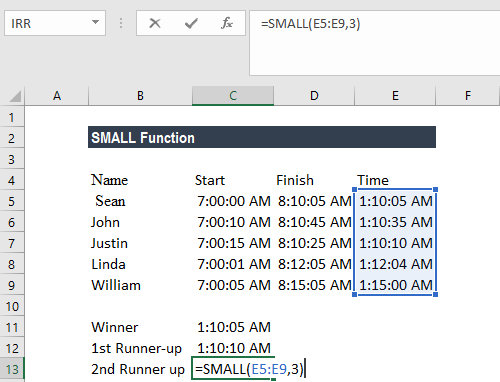We get the following results: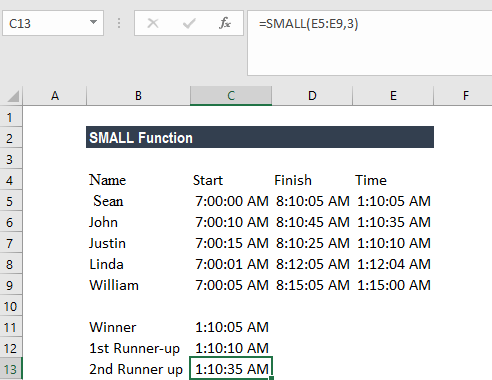### Things to remember about the SMALL Function

1. #NUM! error – Occurs when:
• The given array is empty; or
• The given value of k is less than 1 or greater than the number of values in the given array.
2. #VALUE! error – Occurs if the given k is non-numeric.
3. If n is the number of data points in an array, SMALL(array,1) equals the smallest value, and SMALL(array,n) equals the largest value.
4. Sometimes, the SMALL function will return a wrong value, or will return a #NUM! error, even though the supplied value of k is between 1 and the number of values in the supplied array. This will occur when we provide text values or text representations of numbers within the supplied array. The function ignores text values. Therefore, this problem may arise if the values in the supplied array are text representations of numbers, instead of actual values. We can solve this issue by converting all array values into numeric values.

Thanks for reading CFI’s guide to the Excel SMALL function. By taking the time to learn and master these functions, you’ll significantly speed up your financial modeling. To learn more, check out these additional CFI resources:

• Excel Formulas for Finance